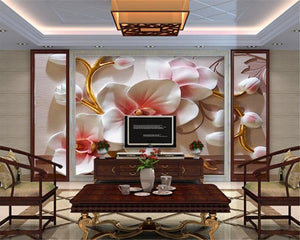# 3D Wallpaper Fashion Floral

\$24.99

\$18.99

3D Wallpaper Fashion Floral

• Decorative floral 3D design
• Flowers bursting out and blooming with gold stems
• Pink, white, and gold colors

Size : 1 square meter

• Price : \$ / Square meter , NOT rolls
• Material available(color) : Silk Cloth
• Not self adhesive - you will need to purchase wallpaper glue

How to place the order?

We have 1m, 5m 10m, 15m, 20m, 25m, 30m, 35m, and 40m available for purchase. Let’s say you need 21 squared meters. You would add 20 squared meters and 1 squared meter to the cart. We will customize the order for 21 square meters. See more examples below.

1 piece=1 square meter

2 square meters = order 2 (1 square meter)

3 square meters = order (3) 1 square meter

7 square meters = order 5 square meter and (2) 1 square meter

12 square meters = order 10 square meters and (2) 1 square meter

How to measure in feet

1 square meter=10.76 square feet

Measure your wall - Width x Height = (w) x (h) in feet.

Multiply the two measurements. Then divide the total by 10.76. See example below.

Wall width measures 9’5” ft. Wall height measure 8’0” ft.

(9’5” x 8’0”) ft = 9.5 x 8 = 76

76 divided by 10.76 = 7.063

The whole number is 7. You would need 7 meters. We suggest you order 8 meters for margin of error(s).

How to measure in centimeters

Measure your wall - Width (cm) x Height (cm)

Multiply the two measurements. Then divide the total by 10,000. See example below.

Wall width measures 670 cm. Wall height measures 510 cm.

670 x 510 = 341,700

341,700 divided by 10,000 = 34.17

The whole number is 34. You would need 34 meters. We suggest you order 35 for margin of error(s).

-------------------------------------------------------------------------

Here are wall size measurements to assist you with the amount of wallpaper you may need.

Quantity 1 square meter = 140cm(W) x 70cm(H) or (4'7" x 2'4") ft
Quantity 2 square meter = 200cm(W) x 100cm(H) or (6'7" x 3'3") ft
Quantity 3 square meter = 220cm(W) x 140cm(H) or (7'3" x 4'7") ft
Quantity 4 square meter = 250cm(W) x 160cm(H) or (8'2" x 5'3") ft
Quantity 5 square meter = 280cm(W) x 180cm(H) or (9'2" x 5'11") ft
Quantity 6 square meter = 300cm(W) x 200cm(H) or (9'10" x 6'7") ft
Quantity 7 square meter = 330cm(W) x 210cm(H) or (10'10" x 6'11") ft
Quantity 8 square meter = 360cm(W) x 230cm(H) or (11'10" x 7'6") ft
Quantity 9 square meter = 380cm(W) x 240cm(H) or (12'5" x 7'10") ft
Quantity 10 square meter = 400cm(W) x 250cm(H) or (13'1" x 8'2") ft
Quantity 11 square meter = 420cm(W) x 260cm(H) or (13'9'' x 8'6'') ft
Quantity 12 square meter = 440cm(W) x 270cm(H) or (14'5" x 8'10") ft
Quantity 13 square meter = 460cm(W) x 280cm(H) or (15'1'' x 9'2'') ft
Quantity 14 square meter = 480cm(W) x 290cm(H) or (15'9'' x 9'6'') ft
Quantity 15 square meter = 500cm(W) x 300cm(H) or (16'5'' x 9'10'') ft
Quantity 16 square meter = 500cm(W) x 320cm(H) or (16'5" x 10'6") ft

Quantity 20 square meter = 580cm(W) x 350cm(H) or (19’0” x 11’5”) ft

Quantity 25 square meter = 640cm(W) x 400cm(H) or (20’11” x 13’1”) ft

Quantity 30 square meter = 660cm(W) x 460cm(H) or (21’7” x 15’1”) ft

Quantity 35 square meter = 680cm(W) x 520cm(H) or (22’3” x 17’0”) ft

Quantity 40 square meter = 690cm(W) x 580cm(H) or (22’7 x 16’4”) ft